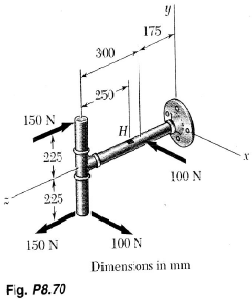# Problem: Several forces are applied to the pipe assembly shown. Knowing that each section of pipe has inner and outer diameters equal to 36 and 42 mm, respectively, determine the normal and shearing stresses at point H located at the top of the outer surface of the pipe.

###### FREE Expert Solution

At point H, we get the normal shear stress from the formula:

$\overline{){{\mathbf{\sigma }}}_{{\mathbf{H}}}{\mathbf{=}}\frac{{\mathbf{M}}_{\mathbf{x}}\mathbf{y}}{{\mathbf{I}}_{\mathbf{x}}}}$

This can be rewritten as:

$\overline{){{\mathbf{\sigma }}}_{{\mathbf{H}}}{\mathbf{=}}\frac{{\mathbf{M}}_{\mathbf{x}}\mathbf{\left(}{\mathbf{d}}_{\mathbf{0}}\mathbf{/}\mathbf{2}\mathbf{\right)}}{\frac{\mathbf{\pi }}{\mathbf{64}}\mathbf{\left(}{{\mathbf{d}}_{\mathbf{0}}}^{\mathbf{4}}\mathbf{-}{{\mathbf{d}}_{\mathbf{i}}}^{\mathbf{4}}\mathbf{\right)}}}$, where Mx is the moment about x, do is the outer diameter of the pipe, and di is the inner diameter of the pipe.

The moments in the x-direction:

93% (120 ratings)###### Problem Details

Several forces are applied to the pipe assembly shown. Knowing that each section of pipe has inner and outer diameters equal to 36 and 42 mm, respectively, determine the normal and shearing stresses at point H located at the top of the outer surface of the pipe.